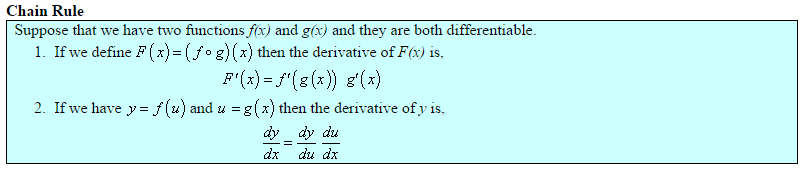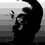# Chain RuleThe product rule tells us how to find the derivative of a product of functions like f(x) • g(x). The composition or “chain” rule tells us how to find the derivative of a composition of functions like f(g(x)). Composition of functions is about substitution – you substitute a value for x into the formula for g, then you substitute the result into the formula for f.

One way to think about composition of functions is to use new variable names. It’s good practice to introduce new variables when they’re convenient, and this is one place where it’s very convenient. So, how do we find the derivative of a composition of functions? We’re trying to find the slope of a tangent line; to do this we take a limit of slopes Δy of Δt (also can be called as (Δy)/(Δt)) secant lines. Here y is a function of x, x is a function of t, and we want to know how y changes with respect to the original variable t. Here again using that intermediate variable x is a big help:

(Δy)/(Δt) = (Δy)/(Δx) times (x)/(Δt)

because when we perform the multiplication, the small change Δx cancels.

The derivative of y with respect to t is “the limit as Δt approaches 0 of (Δy)/(Δt).”

Here is another way of writing the chain rule: d/dx (f◦g)(x) = d/dx (f(g(x))) = f’(g(x)) g’(x)Note by Lew Sterling Jr
6 years, 8 months ago

This discussion board is a place to discuss our Daily Challenges and the math and science related to those challenges. Explanations are more than just a solution — they should explain the steps and thinking strategies that you used to obtain the solution. Comments should further the discussion of math and science.

When posting on Brilliant:

• Use the emojis to react to an explanation, whether you're congratulating a job well done , or just really confused .
• Ask specific questions about the challenge or the steps in somebody's explanation. Well-posed questions can add a lot to the discussion, but posting "I don't understand!" doesn't help anyone.
• Try to contribute something new to the discussion, whether it is an extension, generalization or other idea related to the challenge.

MarkdownAppears as
*italics* or _italics_ italics
**bold** or __bold__ bold
- bulleted- list
• bulleted
• list
1. numbered2. list
1. numbered
2. list
Note: you must add a full line of space before and after lists for them to show up correctly
paragraph 1paragraph 2

paragraph 1

paragraph 2

[example link](https://brilliant.org)example link
> This is a quote
This is a quote
    # I indented these lines
# 4 spaces, and now they show
# up as a code block.

print "hello world"
# I indented these lines
# 4 spaces, and now they show
# up as a code block.

print "hello world"
MathAppears as
Remember to wrap math in $$ ... $$ or $ ... $ to ensure proper formatting.
2 \times 3 $2 \times 3$
2^{34} $2^{34}$
a_{i-1} $a_{i-1}$
\frac{2}{3} $\frac{2}{3}$
\sqrt{2} $\sqrt{2}$
\sum_{i=1}^3 $\sum_{i=1}^3$
\sin \theta $\sin \theta$
\boxed{123} $\boxed{123}$